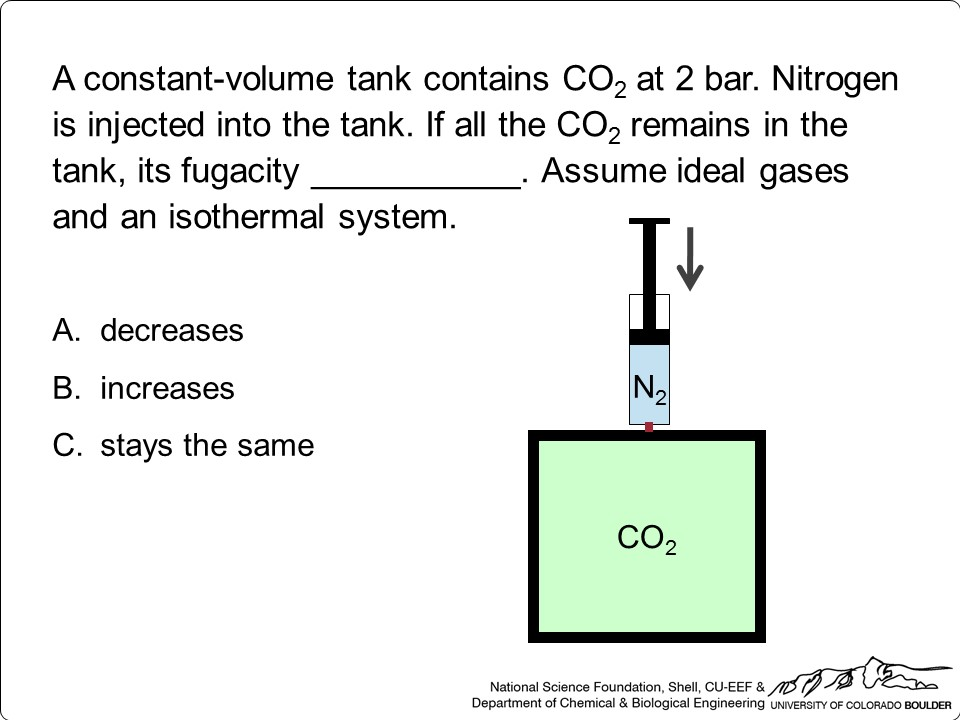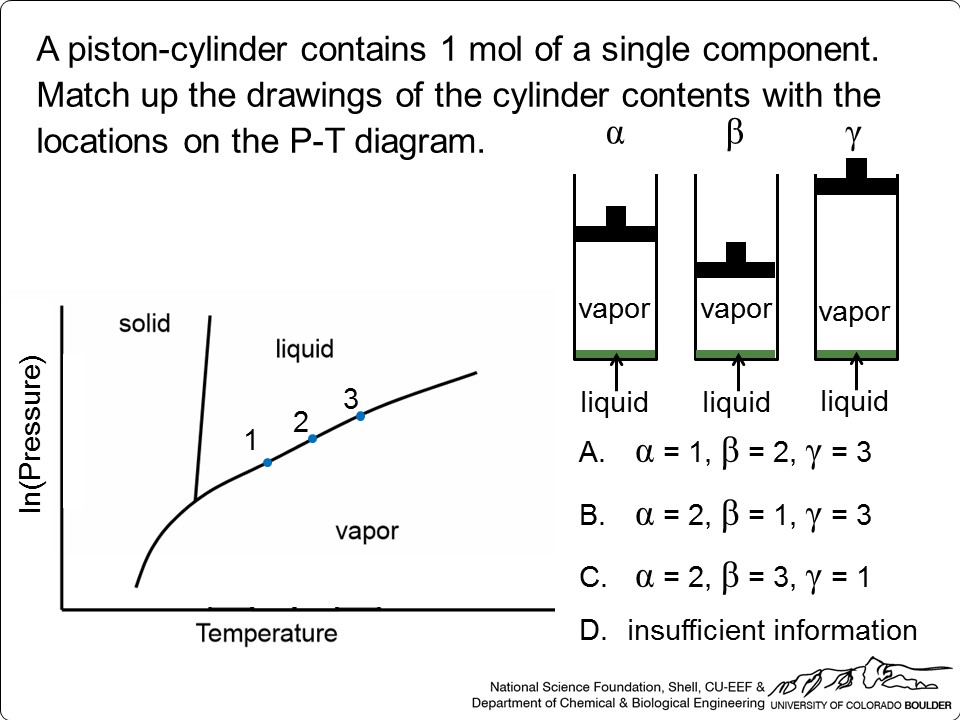# Thermodynamics

## Syllabi, schedules, course notes

• University of California Berkeley: syllabus
• University of Colorado Boulder: Syllabus, schedule, and an all-inclusive digital Microsoft OneNote notebook that uses active learning and a flipped classroom. It contains class notes, ConcepTests, pre-class assignments, recommended screencasts and simulations, homework problems (and solutions), and sample exams (with solutions). Contact LearnChemE@gmail.com to obtain access.

## Screencasts

• LearnChemeE (University of Colorado Boulder): More than 400 short screencast videos for thermodynamics, including examples problems, introduction to topics, software tutorials, exam review problems, and explanations of how to use diagrams.
• Michigan State University: Screencasts organized by the Elliott and Lira textbook

## Interactive Simulations

• Quiz simulations (University of Colorado Boulder): 17 simulations that use a step-by-step procedure in which feedback is provided after the user inputs their solution.

## Interactive Self-Study Modules

• Self-study modules (University of Colorado Boulder): 45 modules that include introductory screencasts (with questions), ConcepTests, interactive simulations, quiz simulations, list of equations and important points, example problem screencasts, and a summary.

## Thermodynamics ConcepTests

Multiple-choice conceptual questions for use in class with student response systems (clickers)

• LearnChemE Instructor Resources (University of Colorado Boulder): More than 1,000 thermodynamics ConcepTests. Contact LearnChemE@gmail.com to obtain access.
• AIChE Concept Warehouse (Tufts University)## Software

• Thermodynamics spreadsheets (Michigan State University): Uses Excel and Peng-Robinson equation of state for single components and mixtures.
• Online homework problems (Michigan State University): 80 randomized problems.
• Polymathplus Solves ODEs, linear equations, and nonlinear equations. Screencasts demonstrating Polymath use can be found here.
• Chemical Engineering AppSuite HD (University of Alabama): A phone app that contains thermophysical property data, steam tables, a period table, units converters, and equation of state solvers. Available for \$0.99 US.
• Equations of state: MATLAB programs for the calculation of phase equilibrium and other thermodynamic properties using different equations of state (classical cubic equations, cubic equations with excess Gibbs energy mixing rules, group contribution equations and SAFT equations).
• Steam tables: spreadsheets with built-in interpolation and screencasts that explain how to use steam tables

CACHE Corporation
P.O. Box 126
Notre Dame, IN 46556
Phone: (574) 631-5687
cache@cache.org